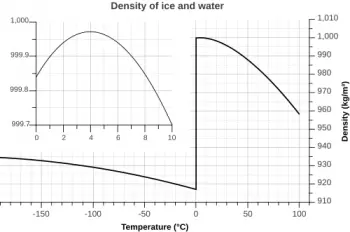# What Is the Density of Water?

The density of water (H2O) depends on many things: the saltpeter content in the water, chemical elements, and dissolved gases it could contain. Moreover, density varies with temperature.

How to calculate the density of an ...
How to calculate the density of an object by water-displacementWe define the density of a substance as the mass per unit volume.

When it is in the liquid state, the maximum density of water is at 4 degrees Celsius, where it is 1000 kg/m3 (one gram per cubic centimeter). When the temperature is above 4 degrees, the density of water decreases.

## What Is the Density of Water in the SI Unit?

The density of pure water in the SI unit is 1000 kg/m3 at its maximum - of 4 degrees Celsius. It means there are one thousand kilograms of water for every cubic meter of space it occupies.

As mentioned, water has its maximum density at 4 degrees Celsius. From this temperature, the water expands when its temperature is increased. At the same time, it also expands when it cools down to zero degrees Celsius. At the freezing point of water (zero degrees Celsius), its density is 999.8 kg/m3

Once it has frozen, the density drops to the density of ice. Ice floats over the water's surface because the lattice arrangement allows water molecules to spread more than in a liquid: 916.8 kg/m3.

Water near the boiling point (100 degrees Celsius) is about 4% less dense than water at four °C.

## What Is the Density of Seawater?

The density of seawater with a salinity index of 35 per thousand is 1027 kg/m3.

Ocean water is a mixture of water and mineral salts, so its density varies depending on the amount of mineral salts present and the temperature. In general, seawater has a higher density than freshwater due to the presence of these minerals.

### Dead Sea Water DensityThe Dead Sea water is known for its high density. Due to evapotranspiration, the water in the Dead Sea evaporates faster than anywhere else in the world, causing the salinity concentration of the water to increase.

We can float in the Dead Sea because the water is denser than the human body.

## How to Calculate the Density of Water?

To measure the density of a liquid such as water, you need to know its weight and volume. Water weight is measured in grams, and the volume is measured in liters.

The density of water is equal to the water's weight divided by the water's volume.

For example, if the water weight is 200 grams and the volume is 1 liter, the density is 200 grams per liter or 200 g/l.

Using a graduated cylinder, we can find the change in density vs. temperature.

Author:

Published: September 20, 2022
Last review: September 20, 2022